# metric driven (1) – aware spike erosion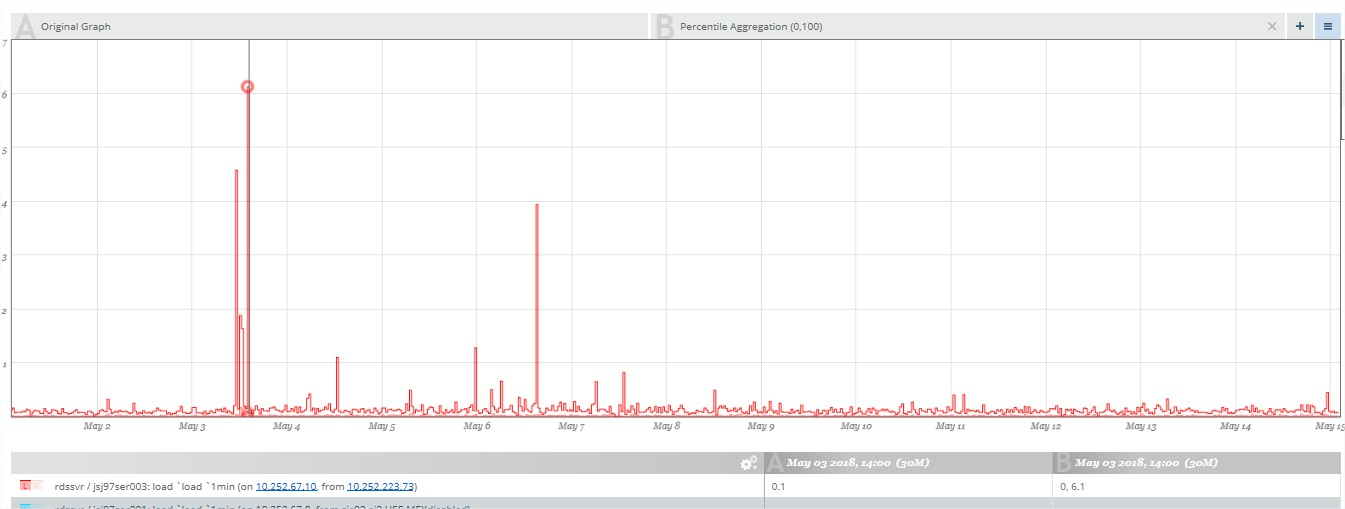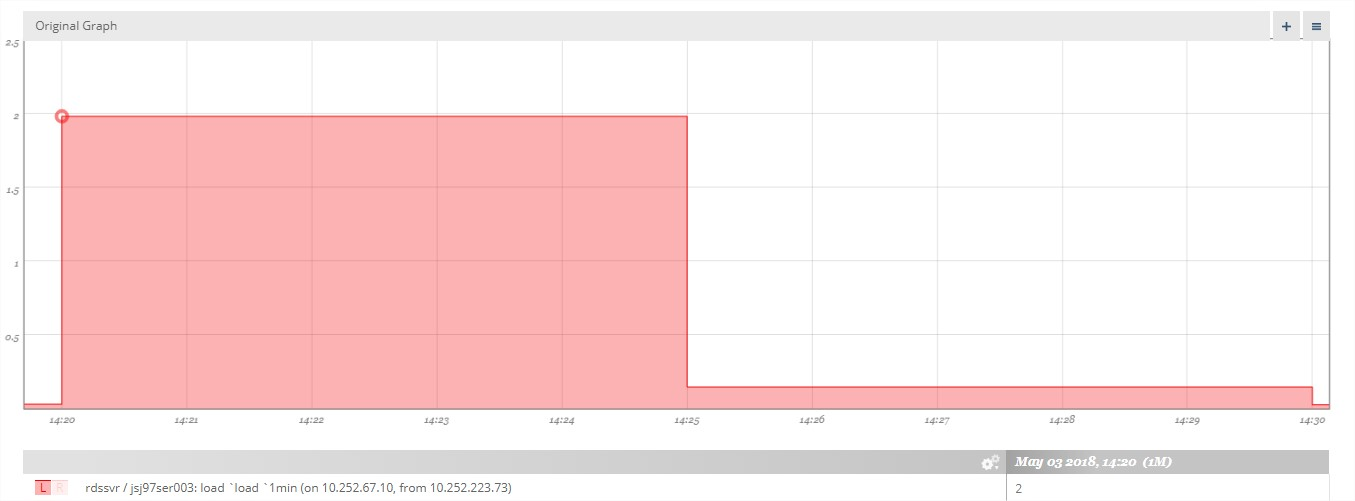• 性能问题：显而易见，“点”越多，绘制的时间越长，性能也越差，可能等几分钟才能等到一幅图渲染完成。例如下图：如此稠密的图，展示就已经耗费很多时间，而实际上稠密的部分并无太多意义：• 显示问题：假设所用的电脑屏幕分辨率是1920 x 1080，同时X轴以时间为单位，当展示大范围的时间范围（例如1年）的时候，则每个肉眼有价值的点是“1年*365天*24小时/1920=4.56天”，而56天的数据范围，要不全部展示（则出现问题1），要不采取一定的策略，仅展示一部分。

（1）对于没有提供辅助方案或者懒于处理数据的用户：

（2）很多metric系统提供了辅助方案：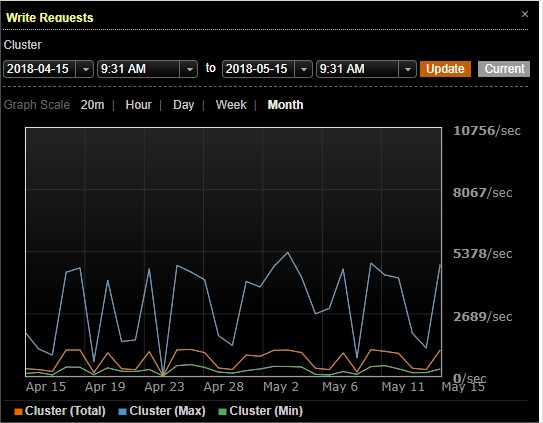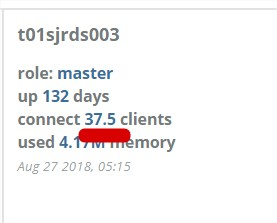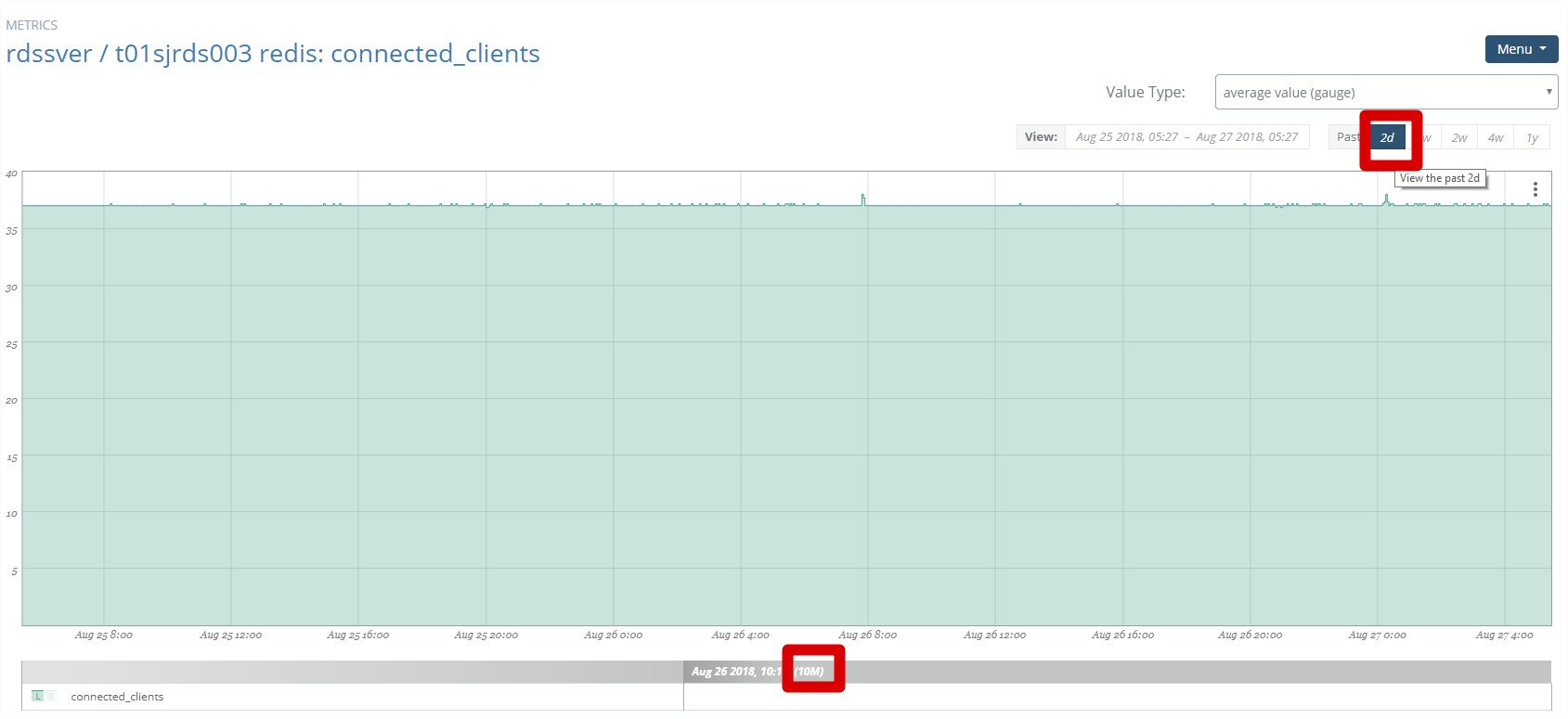```Timer:
name=requests, count=1738812, min=0.489332, max=4.801018, mean=1.072621656614786, stddev=0.38070645373188244, median=1.02622, p75=1.2168807499999998, p95=1.6256607499999998, p98=1.9419521799999988, p99=2.557450750000006, p999=4.771496928000004, mean_rate=0.0, m1=0.0, m5=0.0, m15=0.0, rate_unit=events/millisecond, duration_unit=milliseconds
```

```name=requests, count=1739530, min=0.523682, max=51.885614999999994, mean=1.1354011906614785, stddev=1.6244428387184353, median=1.0495305, p75=1.2338305, p95=1.7184529499999996, p98=1.9595656799999996, p99=2.78311552, p999=50.491461286000174, mean_rate=0.0, m1=0.0, m5=0.0, m15=0.0, rate_unit=events/millisecond, duration_unit=milliseconds
```

99.9%的时间已经达到了50ms, 这里很容易产生疑问，在这么短的时间怎么会产生如此大的差距，那这样的数据是否具有可参考性？

```package com.codahale.metrics;

import static java.lang.Math.min;

/**
* A {@link Reservoir} implementation backed by a sliding window that stores the last {@code N}
* measurements.
*/
public class SlidingWindowReservoir implements Reservoir {
private final long[] measurements;
private long count;

/**
* Creates a new {@link SlidingWindowReservoir} which stores the last {@code size} measurements.
*
* @param size the number of measurements to store
*/
public SlidingWindowReservoir(int size) {
this.measurements = new long[size];
this.count = 0;
}

@Override
public synchronized int size() {
return (int) min(count, measurements.length);
}

@Override
public synchronized void update(long value) {
measurements[(int) (count++ % measurements.length)] = value;
}

@Override
public Snapshot getSnapshot() {
final long[] values = new long[size()];
for (int i = 0; i < values.length; i++) {
synchronized (this) {
values[i] = measurements[i];
}
}
return new Snapshot(values);
}
}
```

## 发布者